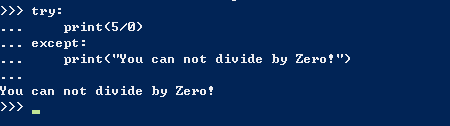﻿ Python基础之异常-编程-比特在线

一、摘要

Python使用被称为异常 的特殊对象来管理程序执行期间发生的错误。每当发生让Python不知所措的错误时，它都会创建一个异常对象。如果你编写了处理该异常的代码，程序将继续运行；如果你未对异常进行处理，程序将停止，并显示一个traceback，其中包含有关异常的报告。

二、异常实操

处理ZeroDivisionError 异常：try-except&try-except-else使用异常避免崩溃：

```print("Give me two numbers, and I'll divide them.") print("Enter 'q' to quit.") while True:     first_number = input("
First number: ")     if first_number == 'q':         break     second_number = input("Second number: ")     if second_number == 'q':         break     answer = int(first_number) / int(second_number)     print(answer)```

`Give me two numbers, and I'll divide them. Enter 'q' to quit. First number: 5 Second number: 0 Traceback (most recent call last):     File "division.py", line 9, in <module>         answer = int(first_number) / int(second_number) ZeroDivisionError: division by zero`

```print("Give me two numbers, and I'll divide them.") print("Enter 'q' to quit.") while True:     first_number = input("
First number: ")     if first_number == 'q':         break     second_number = input("Second number: ")     try:         answer = int(first_number) / int(second_number)     except ZeroDivisionError:         print("You can't divide by 0!")     else:         print(answer)```

`Give me two numbers, and I'll divide them. Enter 'q' to quit. First number: 5 Second number: 0 You can't divide by 0! First number: 5 Second number: 2 2.5 First number: q`

try-except-else 代码块的工作原理大致如下：Python尝试执行try 代码块中的代码；只有可能引发异常的代码才需要放在try 语句中。有时候，有一些仅在try 代码块成功执行时才需要运行的代码；这些代码应放在else 代码块中。except 代码块告诉Python，如果它尝试运行try 代码块中的代码时引发了指定的异常，该怎么办。通过预测可能发生错误的代码，可编写健壮的程序，它们即便面临无效数据或缺少资源，也能继续运行，从而能够抵御无意的用户错误和恶意的攻击。

处理FileNotFoundError 异常：

`filename = 'alice.txt' with open(filename) as f_obj:     contents = f_obj.read()`

`Traceback (most recent call last):     File "alice.py", line 3, in <module>         with open(filename) as f_obj: FileNotFoundError: [Errno 2] No such file or directory: 'alice.txt'`

`filename = 'alice.txt' try:     with open(filename) as f_obj:         contents = f_obj.read()     except FileNotFoundError:         msg = "Sorry, the file " + filename + " does not exist."         print(msg)`

分析文本：

split()函数，看看下边的例子，这个函数干了什么

`>>> title = "Alice in Wonderland" >>> title.split() ['Alice', 'in', 'Wonderland']`

split() 以空格为分隔符将字符串分拆成多个部分，并将这些部分都存储到一个列表中。结果是一个包含字符串中所有单词的列表，虽然有些单词可能包含标点。为计算Alice in Wonderland 包含多少个单词，我们将对整篇小说调用split() ，再计算得到的列表包含多少个元素，从而确定整篇童话大致包含多少个单词

`filename = 'alice.txt' try:     with open(filename) as f_obj:         contents = f_obj.read()     except FileNotFoundError:         msg = "Sorry, the file " + filename + " does not exist."         print(msg)     else:         # 计算文件大致包含多少个单词         words = contents.split()         num_words = len(words)         print("The file " + filename + " has about " + str(num_words) + " words.")`

使用多个文件：

`def count_words(filename):     """计算一个文件大致包含多少个单词"""     try:         with open(filename) as f_obj:             contents = f_obj.read()     except FileNotFoundError:         msg = "Sorry, the file " + filename + " does not exist."         print(msg)     else:         # 计算文件大致包含多少个单词         words = contents.split()         num_words = len(words)         print("The file " + filename + " has about " + str(num_words) + " words.")  filename = 'alice.txt' count_words(filename)`

`filenames = ['alice.txt', 'siddhartha.txt', 'moby_dick.txt', 'little_women.txt'] for filename in filenames:     count_words(filename)`

`def count_words(filename): """计算一个文件大致包含多少个单词"""     try:         --snip--     except FileNotFoundError:         pass     else:         --snip-- filenames = ['alice.txt', 'siddhartha.txt', 'moby_dick.txt', 'little_women.txt'] for filename in filenames:     count_words(filename)`

pass 语句还充当了占位符，它提醒你在程序的某个地方什么都没有做，并且以后也许要在这里做些什么。例如，在这个程序中，我们可能决定将找不到的文件的名称写入到文件missing_files.txt中。用户看不到这个文件，但我们可以读取这个文件，进而处理所有文件找不到的问题